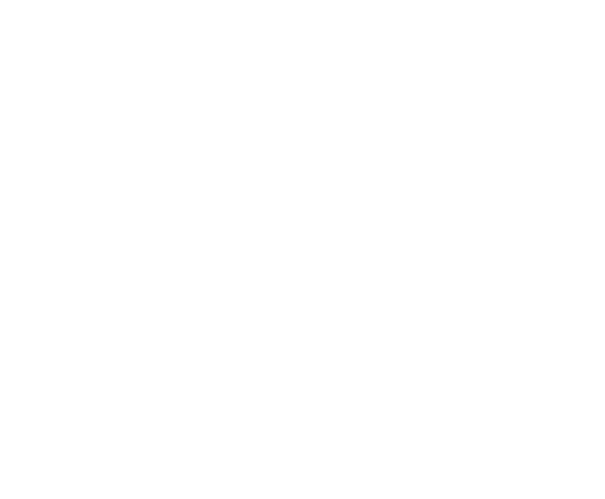# Relation Between Focal Length And Redius Of Curvatur1

﻿

Relation between focal length and redius of curvature

The focal length (f) of a lens is the distance between the center of the lens and the point at which the reflected light, of a beam of light travelling parallel to the center line, meets the center line (principal axis). The radius of curvature (r) is the radius of the lens that forms a complete sphere.Determination of focal length of concave miror by single pin method

• Determine the approximate focal length of the given concave mirror by obtaining on the wall the image of a distant tree.
• Mount the given concave mirror on a stand and fix one pin on the other stand, then place them on the optical bench as shown in the diagram.
•  Now keep the object needle O in front of the mirror M and beyond C. Take a second needle I and place it in between the mirror and the object needle. Move the is needle I, until there is no parallax between the image of O and I on moving the eye from side to side. Measure the distance MO ( u ). Also measure the distance MI ( v ). This gives the observed object and image distance.
•  Very the position of the object bringing it progressively closer to the mirror taking care to see that a real image is obtained in each case. This will be so if object is at a distance greater than the focal length from the mirror. Repeat the above mentioned procedure to find the value of MO and MI in each case. Take atleast six observations in this manner.
•  Plot a graph v vs u. this will be curve. Draw a line OP making an angle of 45o . with either axis and meeting the curve at point P.

Relation between u-v-f

The  formula is a relation between the object distance u , inmage distance v and the focal length from the pole of the concave mirror. The formula is valid for the images in convex mirror and even for the images in lens.  We consider the image formed by aconcave mirror whose focal length is f and whose  radius of curvature is r = 2f.

Let P be the pole of the concave mirror. Let P, F , C be the pole, focucal point , and centre of curvature along principal axis . So, PC = 2PF , as r = 2f.

Let AB  be a vertically standinding object beyond  C on the principal axis.

Then the ray starting from B parallel to  principal axis incident on the mirror at D reflects through the focus F. Let the reflected ray be CFB’ .

The another ray starting from B through the centre C  incident on the mirror at E retraces its path by reflection being normal to the mirror.

Now BE and DF produced meet at B’.

Now drop the perpendicular from B’ to PC to meet at A’.  Drop the perpendicular from D to  PC to meet at G.  Now PF = f , the focal length.

PA = u object distance  from the mirror. PA’ = v the image distance.  Now consider the similar triangles ABC and A’BC.

AB/AB’ = AC/ A’C =( PU-PC)(PC-PA’) = (u-2f)/((2f-v).. .(1)

Consider the similar triangles DFG and A’B’F.

DG/A’B’  = PF/PA’ PF/(PA’-PF)= f/(v-f)… (2)

DG = AB. So (2) could be rewritten as:  AB/A’B’ + f/v ………………..(3).

From (2) and (3), LHS being same , we can equate right sides.

(u-2f)/(2f-v) = f/(v-f).

(u-2f)(v-f) = (2f-v)f.

uv-2fv -fu +2f^2 = 2f^2 -fv  uv = fu +fv  Dvide by uvf;  1/f = 1/v+1/u.

error: Content is protected !!